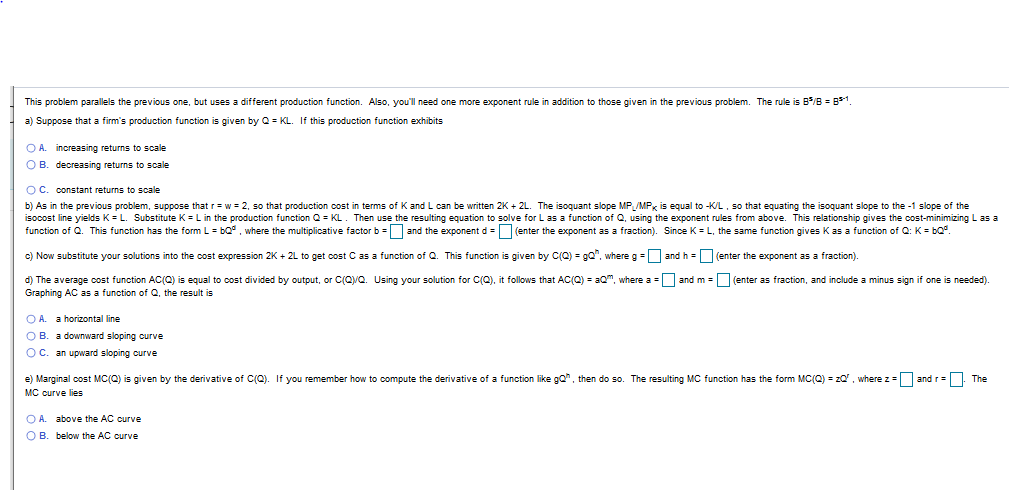# This problem parallels the previous one, but uses a different production function. Also, you'll need one more exponent rule in addition to those given in the previous problem. The rule is B/B B1.a) Suppose that a firm's production function is given by Q KL. If this production function exhibitsO A. increasing returnsO B. decreasing returns to scalescaleO C. constant returns to scaleb) As in the previous problem, suppose that r w 2, so that production cost in terms of K and L can be written 2K 2L. The isoquant slope MP /MP is equal to -KL, so that equating the isoquant slope to the -1 slope of theisocost line yields K L. Substitute K Lin the production function Q KL. Then use the resulting equation to solve for L as a function of Q, using the exponent rules from above. This relationship gives the cost-minimizing L as afunction of Q. This function has the form L bQ where the multiplicative factor b and the exponent d (enter the exponent as a fraction). Since K L, the same function gives K as a function of Q: K bQ.c) Now substitute your solutions into the cost expression 2K+ 2L to get cost C as a function of Q. This function is given by C(Q) gQ", where gand h =(enter the exponent as a fraction)and m (enter as fraction, and include a minus sign if one is needed)d) The average cost function AC(0) is equal to cost divided by output, or C(QQ. Using your solution for C(O), it follows that AC(Q) am, where aGraphing AC as a function of Q, the result isO A.a horizontal lineO B. a downward sloping ourveO C. an upward sloping curvee) Marginal cost MC(Q) is given by the derivative of C(Q). If you remember how to compute the derivative of a function like gQ, then do so. The resulting MC function has the form MC(Q) zQ, where z and rTheMC curve liesO A.above the AC curveO B.below the AC curve

Question
28 views

Part D and ehelp_outlineImage TranscriptioncloseThis problem parallels the previous one, but uses a different production function. Also, you'll need one more exponent rule in addition to those given in the previous problem. The rule is B/B B1. a) Suppose that a firm's production function is given by Q KL. If this production function exhibits O A. increasing returns O B. decreasing returns to scale scale O C. constant returns to scale b) As in the previous problem, suppose that r w 2, so that production cost in terms of K and L can be written 2K 2L. The isoquant slope MP /MP is equal to -KL, so that equating the isoquant slope to the -1 slope of the isocost line yields K L. Substitute K Lin the production function Q KL. Then use the resulting equation to solve for L as a function of Q, using the exponent rules from above. This relationship gives the cost-minimizing L as a function of Q. This function has the form L bQ where the multiplicative factor b and the exponent d (enter the exponent as a fraction). Since K L, the same function gives K as a function of Q: K bQ. c) Now substitute your solutions into the cost expression 2K+ 2L to get cost C as a function of Q. This function is given by C(Q) gQ", where g and h =(enter the exponent as a fraction) and m (enter as fraction, and include a minus sign if one is needed) d) The average cost function AC(0) is equal to cost divided by output, or C(QQ. Using your solution for C(O), it follows that AC(Q) am, where a Graphing AC as a function of Q, the result is O A. a horizontal line O B. a downward sloping ourve O C. an upward sloping curve e) Marginal cost MC(Q) is given by the derivative of C(Q). If you remember how to compute the derivative of a function like gQ, then do so. The resulting MC function has the form MC(Q) zQ, where z and r The MC curve lies O A. above the AC curve O B. below the AC curve fullscreen
check_circle

Step 1

(d) Average total cost is the ratio of total cost and output and is computed as C(Q)/Q, where C(Q) is defined as C(Q) = 2K + 2L. Substituting the value of K = Q1/2 and L = Q1/2 that was found in part (b)

C(Q) = 2Q1/2 + 2 Q1/2

C(Q) = 4Q1/2

AC(Q) = C(Q)/Q

Substituting value of C(Q)

AC(Q) = 4 Q1/2/Q

AC(Q) = 4 Q-1/2

On comparing it with the AC(Q) = aQm, this can be concluded that the value of a = 4 and value  of

m = -1/2.

Step 2

From the graph below this can be concluded that AC curve is downward sl...

### Want to see the full answer?

See Solution

#### Want to see this answer and more?

Solutions are written by subject experts who are available 24/7. Questions are typically answered within 1 hour.*

See Solution
*Response times may vary by subject and question.
Tagged in